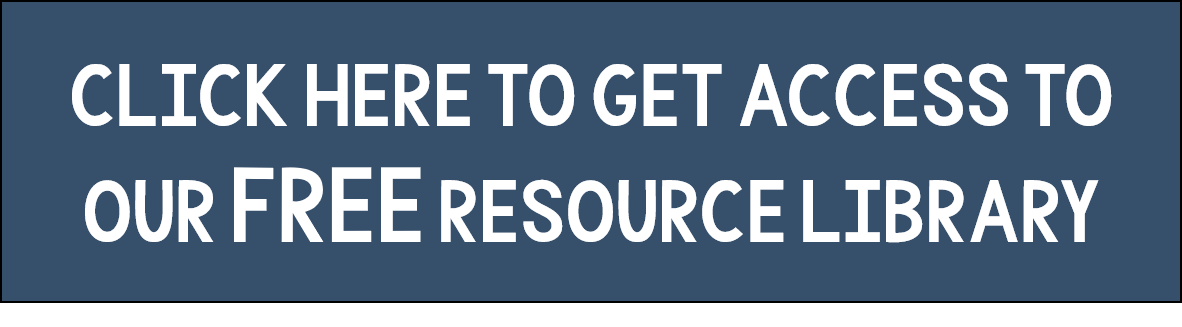# 3 Keys to Become a Better Math Teacher

Teaching MATH
Teaching math is a continuous challenge, it's like reaching a peak only to see another higher peak. There is always more to learn and ways to improve. Here are three tips to become a better math teacher.

Math Teaching Tip 1
Help students make connections between different math topics, especially Algebra and Geometry.  Many people think of mathematics as discrete topics, this is detrimental to students' learning.  As you study mathematics you will learn that math is intricately connected.  Helping students make connections will help them make sense of math and retain the material.

Math Teaching Tip 2
Beware of giving your students algorithms. Students may be able to memorize a few lists of step-by-step algorithms that you give them, but do you expect them to be able to remember ALL the steps for every algorithm?  What about the students that have difficulty memorizing?  I'm not against algorithms, I'm just against giving step-by-step algorithms to your students.  Instead, give them a problem and let them figure it out, then have a discussion with them about what they noticed in their process.  Guide them to discover the algorithm.  Doing this will help them make sense of the mathematics, and internalize the algorithm.

Math Teaching Tip 3
Get writing.  Have your students explain their thinking as much as possible.  Teach them to use mathematical vocabulary as they explain.  Students will often resist writing in math class at first, but be consistent and show good and bad examples so they know what you expect of them.  If you continually require written explanations of their math then your students will internalize the mathematics better.

If you are looking for some resources to get your students writing check out these writing prompts.